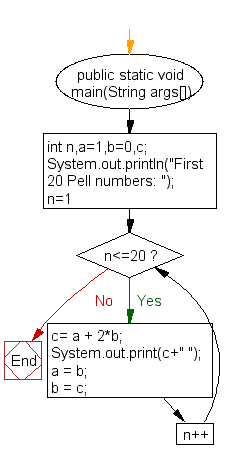﻿ Java exercises: Print the first 15 numbers of the Pell series - w3resource# Java Exercises: Print the first 15 numbers of the Pell series

## Java Numbers: Exercise-25 with Solution

Write a Java program to print the first 15 numbers of the Pell series.

In mathematics, the Pell numbers are an infinite sequence of integers. The sequence of Pell numbers starts with 0 and 1, and then each Pell number is the sum of twice the previous Pell number and the Pell number before that.:
thus, 70 is the companion to 29, and 70 = 2 × 29 + 12 = 58 + 12.
The first few terms of the sequence are :
0, 1, 2, 5, 12, 29, 70, 169, 408, 985, 2378, 5741, 13860,…

Sample Solution:

Java Code:

``````import java.util.Scanner;
public class Example25  {

public static void main(String args[])
{
int n,a=1,b=0,c;
System.out.println("First 20 Pell numbers: ");
for(n=1; n<=20; n++)
{
c= a + 2*b;
System.out.print(c+" ");
a = b;
b = c;
}
}
}
```
```

Sample Output:

```First 20 Pell numbers:
1 2 5 12 29 70 169 408 985 2378 5741 13860 33461 80782 195025 470832 1136689 2744210 6625109 15994428
```

Flowchart:Java Code Editor:

What is the difficulty level of this exercise?

Test your Programming skills with w3resource's quiz.

﻿

## Java: Tips of the Day

How to convert a char array back to a string?

```char[] a = {'h', 'e', 'l', 'l', 'o', ' ', 'w', 'o', 'r', 'l', 'd'};
String b = new String(a);
```

Note however, that this is a very unusual situation: Because String is handled specially in Java, even "foo" is actually a String. So the need for splitting a String into individual chars and join them back is not required in normal code.

Ref: https://bit.ly/39x7Trn